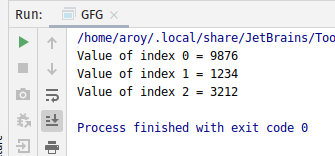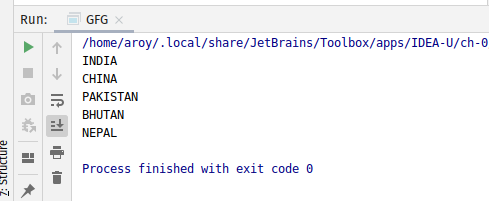# AtomicReferenceArray lazySet() method in Java with Examples

• Last Updated : 03 Jan, 2020

The lazySet() method of a AtomicReferenceArray class is used to set the value of the element at index i to newValue. Both index i and newValue are passed as parameters to the method. This method set the value with memory effects as specified by VarHandle.setRelease(java.lang.Object…) to ensures that prior loads and stores are not reordered after this access.

Syntax:

```public final void lazySet(int i, E newValue)
```

Parameters: This method accepts:

• i which is an index of AtomicReferenceArray to perform the operation,
• newValue which is the new value to set.

Return value: This method returns nothing.

Below programs illustrate the lazySet() method:
Program 1:

 `// Java program to demonstrate``// AtomicReferenceArray.lazySet() method`` ` `import` `java.util.concurrent.atomic.*;`` ` `public` `class` `GFG {``    ``public` `static` `void` `main(String[] args)``    ``{``        ``// create an atomic reference object.``        ``AtomicReferenceArray ref``            ``= ``new` `AtomicReferenceArray(``3``);`` ` `        ``// set some value using lazySet() and print``        ``ref.lazySet(``0``, ``1234``);``        ``ref.lazySet(``1``, ``4322``);``        ``ref.lazySet(``2``, ``2345``);``        ``System.out.println(``"Value of index 0 = "``                           ``+ ref.get(``0``));``        ``System.out.println(``"Value of index 1 = "``                           ``+ ref.get(``1``));``        ``System.out.println(``"Value of index 2 = "``                           ``+ ref.get(``2``));``    ``}``}`

Output:Program 2:

 `// Java program to demonstrate``// AtomicReferenceArray.lazySet() method`` ` `import` `java.util.concurrent.atomic.*;`` ` `public` `class` `GFG {`` ` `    ``public` `static` `void` `main(String[] args)``    ``{`` ` `        ``// create an atomic reference object``        ``AtomicReferenceArray``            ``HIMALAYAN_COUNTRY``            ``= ``new` `AtomicReferenceArray(``5``);`` ` `        ``// set some value``        ``HIMALAYAN_COUNTRY.lazySet(``0``, ``"INDIA"``);``        ``HIMALAYAN_COUNTRY.lazySet(``1``, ``"CHINA"``);``        ``HIMALAYAN_COUNTRY.lazySet(``2``, ``"PAKISTAN"``);``        ``HIMALAYAN_COUNTRY.lazySet(``3``, ``"BHUTAN"``);``        ``HIMALAYAN_COUNTRY.lazySet(``4``, ``"NEPAL"``);`` ` `        ``// print``        ``for` `(``int` `i = ``0``; i < ``5``; i++) {``            ``System.out.println(``                ``HIMALAYAN_COUNTRY.get(i));``        ``}``    ``}``}`

Output:My Personal Notes arrow_drop_up